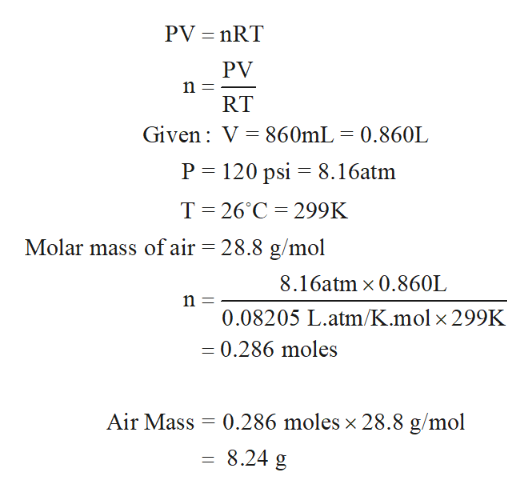# Olympic cyclists fill their tires with helium to make them lighter. Assume the volume of the tire is 860 mL, that is filled to a total pressure of 120 psi, and that the temperature is 26°C. Also assume an average molar mass for air of 28.8 g/mol. Calculate the mass of the air in an air-filled tire? Calculate the mass of helium in a helium-filled tire? What is the mass difference between the two?

Question

Olympic cyclists fill their tires with helium to make them lighter. Assume the volume of the tire is 860 mL, that is filled to a total pressure of 120 psi, and that the temperature is 26°C. Also assume an average molar mass for air of 28.8 g/mol. Calculate the mass of the air in an air-filled tire? Calculate the mass of helium in a helium-filled tire? What is the mass difference between the two?

check_circle

Step 1

First, the moles of air present in ti...help_outlineImage TranscriptionclosePV nRT PV n RT Given V 860mL = 0.860L P 120 psi 8.16atm T 26°C 299K Molar mass of air = 28.8 g/mol 8.16atm x 0.860L n = 0.08205 L.atm/K.mol x 299K =0.286 moles Air Mass 0.286 moles x 28.8 g/mol 8.24 g fullscreen

### Want to see the full answer?

See Solution

#### Want to see this answer and more?

Solutions are written by subject experts who are available 24/7. Questions are typically answered within 1 hour.*

See Solution
*Response times may vary by subject and question.
Tagged in

### Physical Chemistry